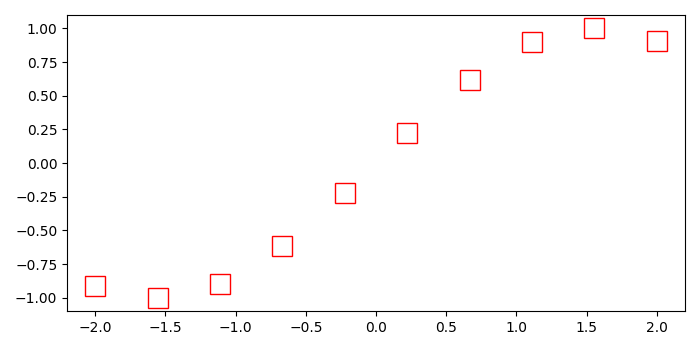# How to make hollow square marks with Matplotlib in Python?

MatplotlibPythonData Visualization

To make hollow square marks with matplotlib, we can use marker 'ks', markerfacecolor='none', markersize=15, and markeredgecolor=red.

## Steps

• Creat x and y data points using numpy.

• Create a figure or activate an existing figure, add an axes to the figure as part of a subplot arrangement.

• Plot x and y data points using plot() method. To make hollow square marks, we can use marker "ks" and markerfacecolor="none", markersize="15" and markeredge color="red".

• To display the figure, use show() method.

## Example

import numpy as np
from matplotlib import pyplot as plt
plt.rcParams["figure.figsize"] = [7.00, 3.50]
plt.rcParams["figure.autolayout"] = True
x = np.linspace(-2, 2, 10)
y = np.sin(x)
fig = plt.figure()
plt.show()